### Home > A2C > Chapter Ch10 > Lesson 10.2.6 > Problem10-155

10-155.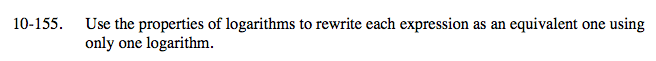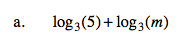Remember the rule logb(N) + logb(M) = logb(NM).

log3 (5m)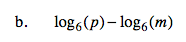$\text{Remember the rule log}_{b}(N) -\text{log}_{b}(M)=\text{log}_{b}\left(\frac{N}{M}\right).$

$\text{log}_{6}\left(\frac{p}{m}\right)$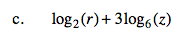Look closely at the bases.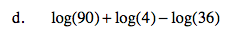See part (a) and (b).

log(10) = 1